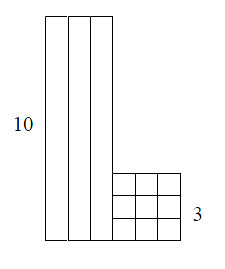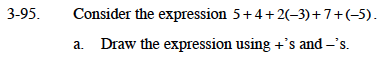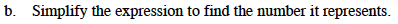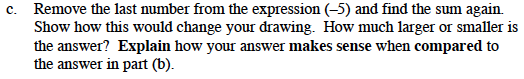### Home > MC1 > Chapter 3 > Lesson 3.4.1 > Problem3-95

3-95.
1. . Consider the expression 5 + 4 + 2(−3) + 7 + (−5). 3-95 HW eTool (CPM). Homework Help ✎

1. Draw the expression using +' s and −' s.

2. Simplify the expression to find the number it represents.

3. Remove the last number from the expression (−5) and find the sum again. Show how this would change your drawing. How much larger or smaller is the answer? Explain how your answer makes sense when compared to the answer in part (b).The first three terms have been drawn for you.

+ + + + +

+ + + +

− − −
− − −The last two terms are drawn below. You can move the drawings from this part and the previous parts to help you make zeroes and determine the answer.

+ + + + + + +

− − − − −You can use the drawings from the parts above to help you with this problem.

Use the following eTool to answer this problem.
Click the link at right for the full version of the eTool: MC1 3-95 HW eTool